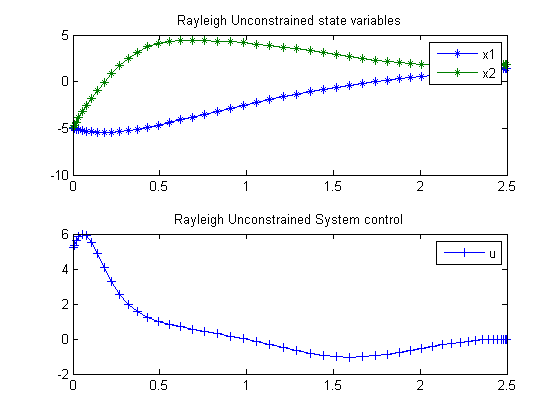91  Rayleigh Unconstrained

Lecture Notes for ECE/MAE 7360, Robust and Optimal Control (part 2) Fall 2003, Jinsong Liang, Nov. 20, 2003 Utah State University at Logan

91.1  Problem Formulation

Find u over t in [0; t_F ] to minimize

J =
 2.5 0
x12 + u2 dt

subject to:

 dx1 dt
= x2
 dx2 dt
= −x1+(1.4−0.14*x22)*x2+4*u
 x1(0) = −5
 x2(0) = −5

Reference: 

91.2  Problem setup

toms t
p = tomPhase('p', t, 0, 2.5, 50);
setPhase(p);

tomStates x1 x2
tomControls u

% Initial guess
x0 = {icollocate({x1 == -5; x2 == -5})
collocate(u == 0)};

% Box constraints
cbox = {-100 <= icollocate(x1) <= 100
-100 <= icollocate(x2) <= 100
-100 <= collocate(u) <= 100};

% Boundary constraints
cbnd = initial({x1 == -5; x2 == -5});

% ODEs and path constraints
ceq = collocate({dot(x1) == x2
dot(x2) == -x1+(1.4-0.14*x2.^2).*x2+4*u});

% Objective
objective = integrate(x1.^2+u.^2);

91.3  Solve the problem

options = struct;
options.name = 'Rayleigh Unconstrained';
solution = ezsolve(objective, {cbox, cbnd, ceq}, x0, options);
t  = subs(collocate(t),solution);
x1 = subs(collocate(x1),solution);
x2 = subs(collocate(x2),solution);
u  = subs(collocate(u),solution);
Problem type appears to be: qpcon
Starting numeric solver
===== * * * =================================================================== * * *
TOMLAB - Tomlab Optimization Inc. Development license  999001. Valid to 2011-02-05
=====================================================================================
Problem: ---  1: Rayleigh Unconstrained         f_k      29.376079656023496000
sum(|constr|)      0.000000003740997838
f(x_k) + sum(|constr|)     29.376079659764493000
f(x_0)     62.499999999999986000

Solver: snopt.  EXIT=0.  INFORM=1.
SNOPT 7.2-5 NLP code
Optimality conditions satisfied

FuncEv    1 ConstrEv   47 ConJacEv   47 Iter   36 MinorIter  144
CPU time: 0.250000 sec. Elapsed time: 0.266000 sec.

91.4  Plot result

subplot(2,1,1)
plot(t,x1,'*-',t,x2,'*-');
legend('x1','x2');
title('Rayleigh Unconstrained state variables');

subplot(2,1,2)
plot(t,u,'+-');
legend('u');
title('Rayleigh Unconstrained System control');